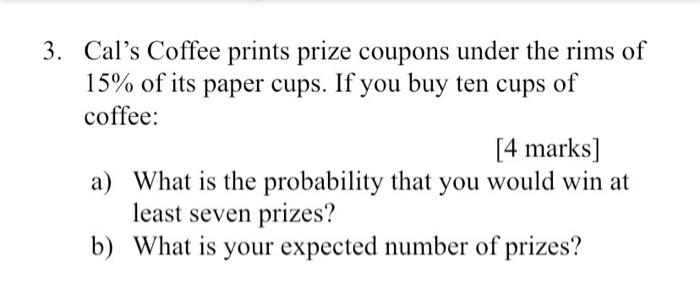# Question DISCRETE DISTRUBUTIONS. COMPLETE THE ENTIRE PARTS. I WILL LEAVE GOOD REVIEW 3. Cal's Coffee prints prize coupons under the rims of 15% of its paper cups. If you buy ten cups of coffee: [4 marks] a) What is the probability that you would win at least seven prizes? b) What is your expected number of prizes?L3VAH6 The Asker · Probability and Statistics
DISCRETE DISTRUBUTIONS. COMPLETE THE ENTIRE PARTS. I WILL LEAVE GOOD REVIEWTranscribed Image Text: 3. Cal's Coffee prints prize coupons under the rims of 15% of its paper cups. If you buy ten cups of coffee: [4 marks] a) What is the probability that you would win at least seven prizes? b) What is your expected number of prizes?
More
Transcribed Image Text: 3. Cal's Coffee prints prize coupons under the rims of 15% of its paper cups. If you buy ten cups of coffee: [4 marks] a) What is the probability that you would win at least seven prizes? b) What is your expected number of prizes?